# 计算机视觉"新"范式: Transformer

## Transformer

Transformer详解

The Illustrated Transformer
https://jalammar.github.io/illustrated-transformer/

1. Encoder-Decoder

Transformer结构可以表示为Encoder和Decoder两个部分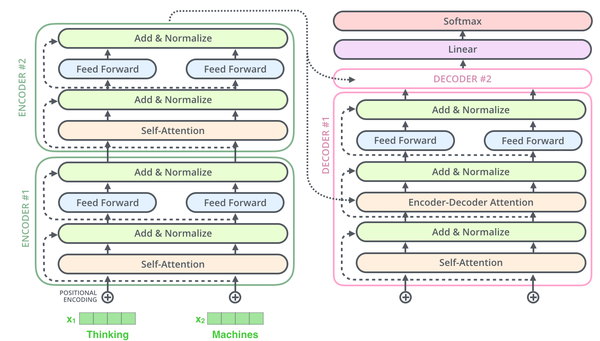Scaled Dot-Product Attention公式：

$$\operatorname{Attention}(Q , K , V ) = \operatorname { softmax } \left( \frac { Q K ^ { T } } { \sqrt { d _ { k } } } \right) V$$

$$\operatorname{MultiHead}(Q, K , V ) = \text { Concat } \left( \text { head } { 1 } , \ldots , \text { head } { \mathrm { h } } \right) W ^ { O }$$

Feed-Forward Network公式：

$$\operatorname { FFN } ( x ) = \max \left( 0 , x W { 1 } + b { 1 } \right) W { 2 } + b { 2 }$$

2. Positional Encoding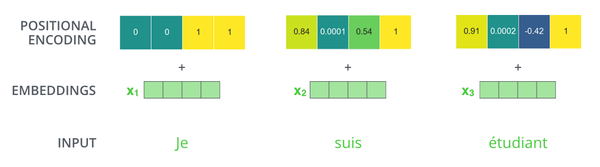\begin{aligned} P E { ( p o s , 2 i ) } & = \sin \left( p o s / 10000 ^ { 2 i / d { \text {nodel } } } \right) \ P E { ( p o s , 2 i + 1 ) } & = \cos \left( p o s / 10000 ^ { 2 i / d { \text {model } } } \right) \end{aligned}

$pos$ 是位置， $i$ 是维数， $d_{model}$ 是输入单词的嵌入向量维度。

3. Self-Attention

3.1 Scaled Dot-Product Attention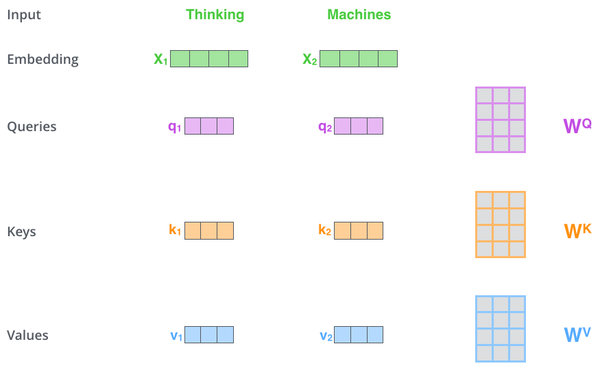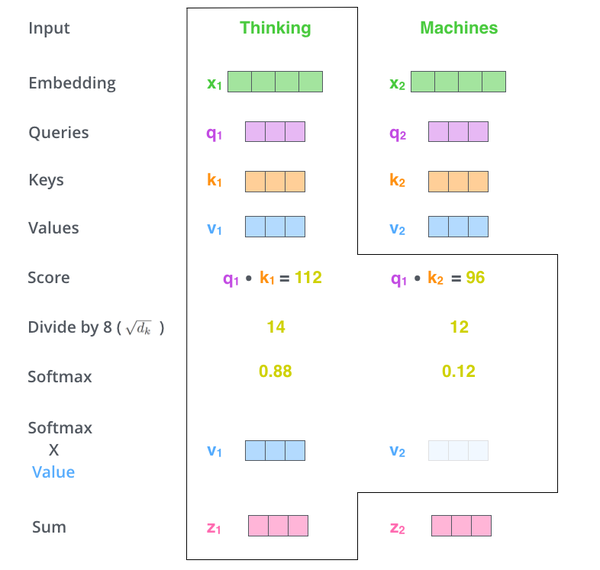1. 每个输入单词转化成嵌入向量。
2. 根据嵌入向量得到$q$，$k$，$v$三个向量。
3. 通过向量计算 ： 。
4. 对 $q$ ，$k$进行归一化，即除以$\sqrt { d _ { k } }$。
5. 通过$softmax$激活函数计算$score$。
6. 点乘Value值$v$，得到每个输入向量的评分$v$。
7. 所有输入向量的评分$v$之和为$z$：$z = \sum v$。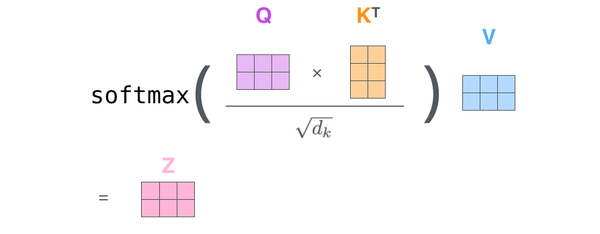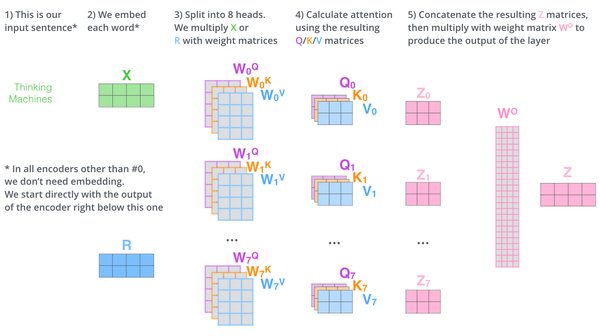1. 将数据 $X$ 分别输入到8个不同的Scaled Dot-Product Attention中，得到8个加权后的特征矩阵 $Z _ { i } , i \in { 1,2 , \ldots , 8 }$ 。
2. 将8个 $Z$ 按列拼成一个大的特征矩阵。
3. 特征矩阵经过一层全连接得到输出 $Z$ 。

## ViT

ViT将Transformer巧妙的应用于图像分类任务，更少计算量下性能跟SOTA相当。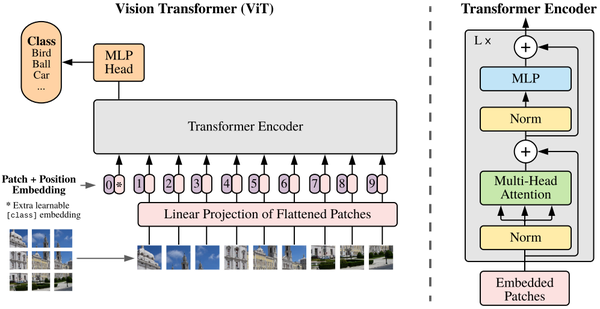Vision Transformer(ViT)将输入图片拆分成16x16个patches，每个patch做一次线性变换降维同时嵌入位置信息，然后送入Transformer，避免了像素级attention的运算。类似BERT[class]标记位的设置，ViT在Transformer输入序列前增加了一个额外可学习的[class]标记位，并且该位置的Transformer Encoder输出作为图像特征。

\begin{aligned} \mathbf { z } { 0 } & = \left[ \mathbf { x } { \text {class } } ; \mathbf { x } { p } ^ { 1 } \mathbf { E } ; \mathbf { x } { p } ^ { 2 } \mathbf { E } ; \cdots ; \mathbf { x } { p } ^ { N } \mathbf { E } \right] + \mathbf { E } { \text {pos} } , & \mathbf { E } & \in \mathbb { R } ^ { \left( P ^ { 2 } \cdot C \right) \times D } , \mathbf { E } { \text {pos} } \in \mathbb { R } ^ { ( N + 1 ) \times D } \ \mathbf { z } { \ell } ^ { \prime } & = \operatorname { MSA } \left( \mathrm { LN } \left( \mathbf { z } { \ell - 1 } \right) \right) + \mathbf { z } { \ell - 1 } , & & \ell = 1 \ldots L \ \mathbf { z } { \ell } & = \operatorname { MLP } \left( \operatorname { LN } \left( \mathbf { z } { \ell } ^ { \prime } \right) \right) + \mathbf { z } { \ell } ^ { \prime } , & & \ell = 1 \ldots L \ \mathbf { y } & = \operatorname { LN } \left( \mathbf { z } { L } ^ { 0 } \right) \end{aligned}

ViT舍弃了CNN的归纳偏好问题，更加有利于在超大规模数据上学习知识，即大规模训练优归纳偏好，在众多图像分类任务上直逼SOTA。

## DETR

DETR使用set loss function作为监督信号来进行端到端训练，然后同时预测所有目标，其中set loss function使用bipartite matching算法将pred目标和gt目标匹配起来。直接将目标检测任务看成set prediction问题，使训练过程变的简洁，并且避免了anchor、NMS等复杂处理。

DETR主要有两个部分：architecture和set prediction loss。

1. Architecture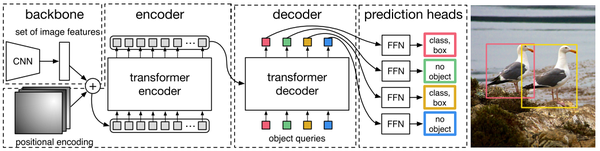DETR先用CNN将输入图像embedding成一个二维表征，然后将二维表征转换成一维表征并结合positional encoding一起送入encoder，decoder将少量固定数量的已学习的object queries(可以理解为positional embeddings)和encoder的输出作为输入。最后将decoder得到的每个output embdding传递到一个共享的前馈网络(FFN)，该网络可以预测一个检测结果(包括类和边框)或着“没有目标”的类。

1.1 Transformer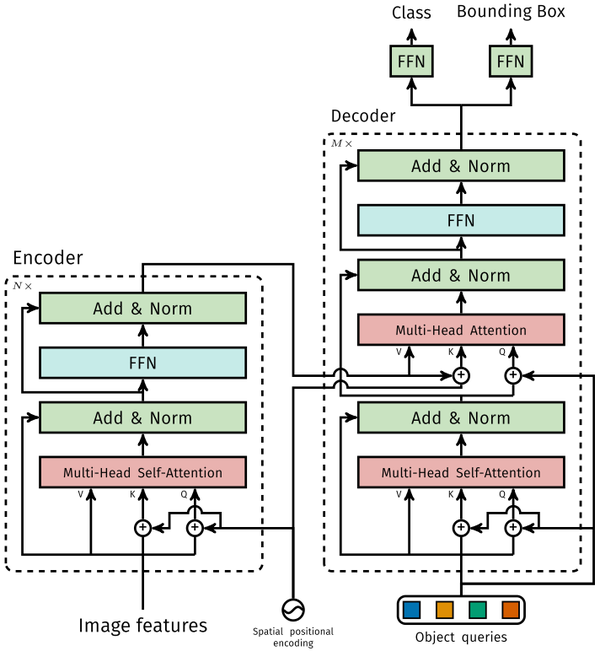1.1.1 Encoder

1.1.2 Decoder

1.1.3 FFN

FFN由3层perceptron和一层linear projection组成。FFN预测出box的归一化中心坐标、长、宽和class。

DETR预测的是固定数量的$N$个box的集合，并且$N$通常比实际目标数要大的多，所以使用一个额外的空类来表示预测得到的box不存在目标。

2. Set prediction loss

DETR模型训练的主要困难是如何根据gt衡量预测结果(类别、位置、数量)。DETR提出的loss函数可以产生pred和gt的最优双边匹配(确定pred和gt的一对一关系)，然后优化loss。

$$\hat { \sigma } = \underset { \sigma \in \mathfrak { S } { N } } { \arg \min } \sum { i } ^ { N } \mathcal { L } { \operatorname { match } } \left( y { i } , \hat { y } _ { \sigma ( i ) } \right)$$

hungarian loss公式如下：

$$\mathcal { L } { \text {Hungarian } } ( y , \hat { y } ) = \sum { i = 1 } ^ { N } \left[ - \log \hat { p } { \hat { \sigma } ( i ) } \left( c { i } \right) + \mathbb { 1 } { \left{ c { i } \neq \varnothing \right} } \mathcal { L } { \text {box } } \left( b { i } , \hat { b } _ { \hat { \sigma } } ( i ) \right) \right]$$

$$\mathcal { L } { \mathrm { box } } \left( b { i } , \hat { b } { \sigma ( i ) } \right)=\mathcal { L } { \mathrm { iou } } \left( b { i } , \hat { b } { \sigma ( i ) } \right) + \lambda { \mathrm { L } 1 } \left| b { i } - \hat { b } { \sigma ( i ) } \right| { 1 }$$

ViT和DETR两篇文章的实验和可视化分析很有启发性，感兴趣的可以仔细看看\~\~

### Deformable DETR

Deformable DETR将DETR中的attention替换成Deformable Attention，使DETR范式的检测器更加高效，收敛速度加快10倍。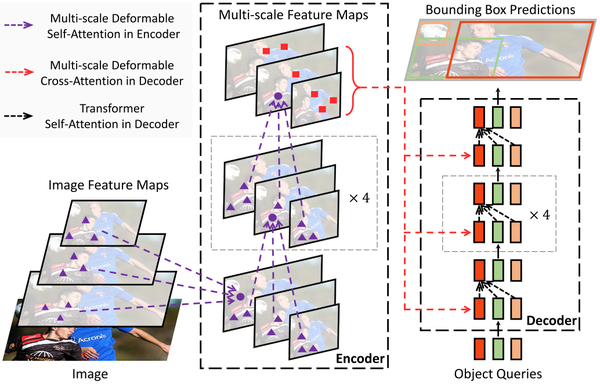Deformable DETR提出的Deformable Attention可以可以缓解DETR的收敛速度慢和复杂度高的问题。同时结合了deformable convolution的稀疏空间采样能力和transformer的关系建模能力。Deformable Attention可以考虑小的采样位置集作为一个pre-filter突出所有feature map的关键特征，并且可以自然地扩展到融合多尺度特征，并且Multi-scale Deformable Attention本身就可以在多尺度特征图之间进行交换信息，不需要FPN操作。

1. Deformable Attention Module

$$\operatorname{MultiHeadAttn}(\left. \boldsymbol { z } { q } , \boldsymbol { x } \right) = \sum { m = 1 } ^ { M } \boldsymbol { W } { m } \left[ \sum { k \in \Omega { k } } A { m q k } \cdot \boldsymbol { W } { m } ^ { \prime } \boldsymbol { x } { k } \right]$$

$$\operatorname{Attn}\left( \boldsymbol { z } { q } , \boldsymbol { x } \right) = \sum { m = 1 } ^ { M } \boldsymbol { W } { m } \left[ \sum { k = 1 } ^ { K } A { m q k } \cdot \boldsymbol { W } { m } ^ { \prime } \boldsymbol { x } \right]$$

$$\operatorname{DeformAttn}\left( \boldsymbol { z } { q } , \boldsymbol { p } { q } , \boldsymbol { x } \right) = \sum { m = 1 } ^ { M } \boldsymbol { W } { m } \left[ \sum { k = 1 } ^ { K } A { m q k } \cdot \boldsymbol { W } { m } ^ { \prime } \boldsymbol { x } \left( \boldsymbol { p } { q } + \Delta \boldsymbol { p } _ { m q k } \right) \right]$$

2. Multi-scale Deformable Attention Module

Deformable Attention可以很自然地扩展到多尺度的feature maps。$\left{ \boldsymbol { x } ^ { l } \right} { l = 1 } ^ { L }$表示为输入的多尺度feature maps，$\boldsymbol { x } ^ { l } \in \mathbb { R } ^ { C \times H { l } \times W { l } }$。 $\hat { \boldsymbol { p } } { q } \in [ 0,1 ] ^ { 2 }$ 表示为每个query元素$q$的参考点 $p$ 的归一化坐标。Deformable DETR 的Multi-scale Deformable Attention公式表示为：

$$\operatorname{MSDeformAttn}\left( \boldsymbol { z } { q } , \hat { \boldsymbol { p } } { q } , \left{ \boldsymbol { x } ^ { l } \right} { l = 1 } ^ { L } \right) = \sum { m = 1 } ^ { M } \boldsymbol { W } { m } \left[ \sum { l = 1 } ^ { L } \sum { k = 1 } ^ { K } A { m l q k } \cdot \boldsymbol { W } { m } ^ { \prime } \boldsymbol { x } ^ { l } \left( \phi { l } \left( \hat { \boldsymbol { p } } { q } \right) + \Delta \boldsymbol { p } { m l q k } \right) \right]$$

3. Deformable Transformer Encoder

4. Deformable Transformer Decoder

Decoder中有cross-attention和self-attention两种注意力。这两种注意力的query元素都是object queries。在cross-attention中，object queries从feature maps中提取特征，而key元素是encoder输出的feature maps。在self-attention中，object queries之间相互作用，key元素也是object queries。因为Deformable Attention是用于key元素的feature maps特征提取的，所以decoder部分，deformable attention只替换cross-attention。

## 复杂度分析

Convolution复杂度

• 为了保证输入和输出在第一个维度都相同，故需要对输入进行padding操作，因为这里kernel size为$k$(实际kernel的形状为$k \times k \times d$)。
• 大小为$k \times k$的卷积核一次运算复杂度为$O(k^2d)$，一共做了$H\times W$次，故复杂度为$O(HWk^2d)$。
• 为了保证第三个维度相等，故需要$d$个卷积核，所以卷积操作的时间复杂度为$O(HWK^2d^2)$。

Self-Attention复杂度

$$\operatorname{Attention}(Q , K , V ) = \operatorname { softmax } \left( \frac { Q K ^ { T } } { \sqrt { d _ { k } } } \right) V$$

• $Q,K,V$的计算复杂度为$n \times d$。
• 相似度计算$QK^T$：$n \times d$与$d \times n$运算，得到$n \times n$矩阵，复杂度为$O(n^2d)$。
• $softmax$ 计算：对每行做 $softmax$ ，复杂度为$O(n)$，则n行的复杂度为$O(n^2)$。
• 加权和：$n \times n$与$n \times d$运算，得到$n \times d$矩阵，复杂度为$O(n^2d)$。
• 故最后self-attention的时间复杂度为$O(n^2d)$。

Transformer

Self-Attention的复杂度为$O(n^2d)$。

ViT

$$N = H \times W/P^2$$

Self-Attention的复杂度为$O(H^2W^2d/P^4)$。

DETR

$$n = H \times W$$

Self-Attention的复杂度为$O(H^2W^2d)$。

Deformable DETR

Self-Attention的复杂度为$O(HWd^2)$。

## 几个问题

$Q,K,V$如何理解\? 为什么不使用相同的$Q$$K$

1. 从点乘的物理意义上讲，两个向量的点乘表示两个向量的相似度。

2. $Q,K,V$的物理意义是一样的，都表示同一个句子中不同token组成的矩阵。矩阵中的每一行，是表示一个token的word embedding向量。假设一个句子“Hello, how are you\?”长度是6，embedding维度是300，那么$Q,K,V$都是(6，300)的矩阵。

transformer中为什么使用不同的K 和 Q， 为什么不能使用同一个值？

https://medium.com/dissecting-bert/dissecting-bert-part-1-d3c3d495cdb3

Xinlong Wang：CNN是怎么学到图片内的绝对位置信息的?
https://zhuanlan.zhihu.com/p/99766566

ViT为什么要增加一个[CLS]标志位\? 为什么将[CLS]标志位对应的向量作为整个序列的语义表示\?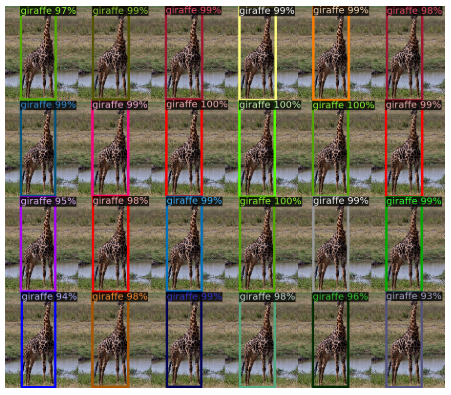https://liam.page/2016/04/03/Hungarian-algorithm-in-the-maximum-matching-problem-of-bigraph/
ZihaoZhao：带你入门多目标跟踪（三）匈牙利算法\&KM算法
https://zhuanlan.zhihu.com/p/62981901

BETR的positional embedding、object queries和slot三者之间有何关系？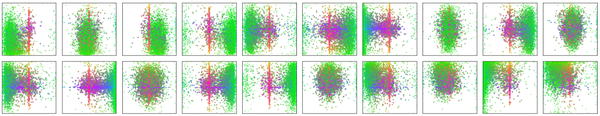DETR可视化decoder预测得到的20个slot。可以观察到每个slot学习到了特定区域的尺度大小。Object queries从这个角度看，其实有点像Faster-RCNN等目标检测器的anchor，结合encoder的positional embedding信息让每个slot往学习到的特定区域去寻找目标。

Transformer相比于CNN的优缺点\?

Transformer关注全局信息，能建模更加长距离的依赖关系，而CNN关注局部信息，全局信息的捕捉能力弱。

Transformer避免了CNN中存在的归纳偏好问题。

Transformer复杂度比CNN高，但是ViT和Deformable DETR给出了一些解决方法来降低Transformer的复杂度。

## 总结

Transformer给图像分类和目标检测方向带来了巨大革新，分割、跟踪、视频等方向也不远了吧

NLP和CV的关系变的越来越有趣了，虽然争议很大，但是试想一下，NLP和CV两个领域能用一种范式来表达，该有多可怕，未来图像和文字是不是可以随心所欲的转来转去？可感知可推理的强人工智能是不是不远了？(想想就好)

Reference

Attention Is All You Need

An Image is Worth 16*16 Words: Transformers for Image Recognition at Scale

End-to-End Object Detection with Transformers

Deformable DETR: Deformable Transformers for End-to-End Object Detection